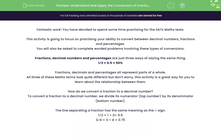# Understand and Apply the Conversion of Fractions, Decimals and Percentages 3

In this worksheet, students will be asked to convert between fractions, decimals and percentages. They will also be asked to solve worded problems involving such conversions.This content is premium and exclusive to EdPlace subscribers.Key stage:  KS 2

Curriculum topic:   Exam-style Questions: SATs Maths

Curriculum subtopic:   Exam-Style Questions: Decimal Conversion

Difficulty level:#### Worksheet Overview

Fantastic work! You have decided to spend some time practising for the SATs maths tests.

This activity is going to focus on practising your ability to convert between decimal numbers, fractions and percentages.

You will also be asked to complete worded problems involving these types of conversion.

Fractions, decimal numbers and percentages are just three ways of saying the same thing:

1⁄2 = 0.5 = 50%

Fractions, decimals and percentages all represent parts of a whole.

All three of these maths terms look quite different but don't worry, this activity is a great way for you to learn about the relationship between them.

How do we convert between fractions and decimals?

To convert a fraction to a decimal number, we divide its numerator (top number) by its denominator (bottom number).

The line separating a fraction has the same meaning as the ÷ sign.

1⁄2 = 1 ÷ 2= 0.5

3⁄4 = 3 ÷ 4 = 0.75Remember to use the bus stop method of division to help you solve the sum.

To convert a decimal into a fraction, you need to make the denominator 10, 100 or 1,000 etc depending on how many decimal places there are in the decimal.

0.36 means 36 hundredths so it becomes 36/100 which can then be cancelled down to 9/25

0.7 means 7 tenths so it becomes 7/10

How do we convert between percentages and fractions?

To convert a percentage into a fraction, you simply divide the percentage by 100:

60% to a fraction becomes 60 ÷ 100 or simply 60/100

This can then be simplified to 6/10

To convert a fraction into a percentage, you multiply the fraction by 100:

5/20 to a percentage becomes 5/20 x 100: Divide 100 by the denominator of 20:  100 ÷ 20 = 5.

Then multiply that answer by the numerator: 5 x 5 = 25

How do we convert between decimal numbers and percentages?

To convert a decimal number to a percentage, we multiply the decimal number by 100.

Remember, when you multiply by 100, move all of the digits two places to the left.

0.75 x 100% = 75.0% = 75%

Look at the place value chart below to see the movement of the digits.

 Hundreds Tens Ones . Tenths Hundredths 0 . 7 5 7 5 . 0

To convert a percentage to a decimal number, we divide the decimal number by 100.

Remember, when you divide by 100, move all of the digits two places to the right.

75% ÷ 100 = 0.75

Look at the place value chart below to see the movement of the digits.

 Hundreds Tens Ones . Tenths Hundredths 7 5 . 0 0 . 7 5

Over to you now to have a go at some practice questions...good luck!

### What is EdPlace?

We're your National Curriculum aligned online education content provider helping each child succeed in English, maths and science from year 1 to GCSE. With an EdPlace account you’ll be able to track and measure progress, helping each child achieve their best. We build confidence and attainment by personalising each child’s learning at a level that suits them.

Get started